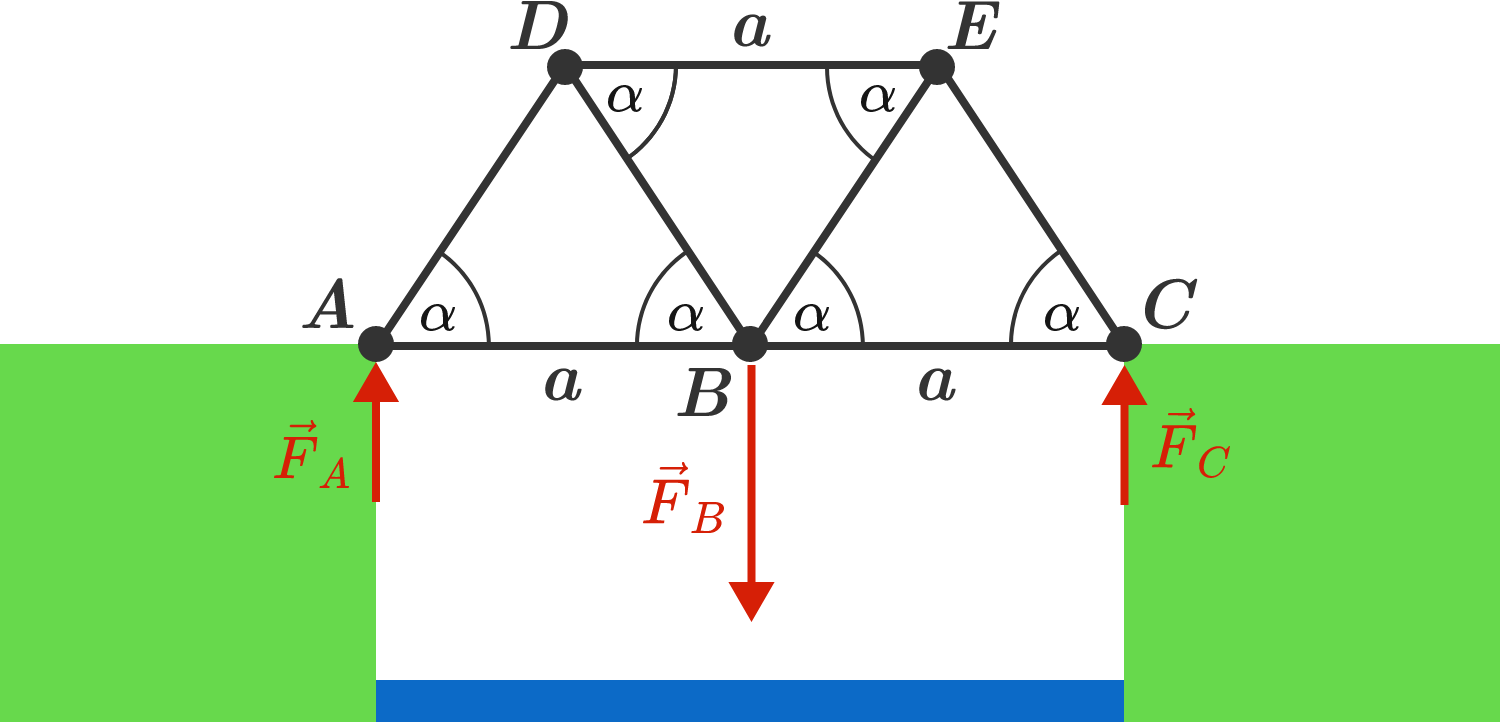# Poly BridgeA simple bridge of three triangular elements spans a river and is carried by the foundation at $A$ and $C$ in the diagram. The connecting lines between the points correspond to steel girders, each of which can bear a maximum tensile and compressive load of 10 tons.

What is the minimum angle $\alpha$ needed so that a truck with a weight of 10 tons can safely cross the bridge?

Hints:

• Assume that the weight load of the truck is concentrated at point $B$, so here a force of $\vec F_B = (10 \,\text{tons})\,\cdot \vec g$ acts on the bridge.
• The bridge is held by the opposing forces $\vec F_A = \vec F_C = - \frac{1}{2} \vec F_B$ on points $A$ and $C$. Calculate the forces acting along the steel girders.

Note: for every point of the polygon, the sum of all forces must be zero.

×

Problem Loading...

Note Loading...

Set Loading...